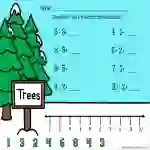# December Math PowerPoint, Addition and SubtractionAttributes
Subject

Numbers

Types

Activities

Editable
NoAre you looking for digital math for December? These fun and interactive December Math PowerPoint, Addition and Subtraction will keep your students engaged and learning!

This December Math PowerPoint, Addition and Subtraction include 8 slides with number line addition and subtraction through 20 and ten frame addition and subtraction through 10. Each slide includes a set of 8 additions or subtractions in addition to the number line with options of answers to pick from. This December Math PowerPoint, Addition and Subtraction includes a total of 10 slides, hence 80 problems of addition and subtraction in total.

These addition and subtraction slides are perfect to use for math centers, reinforcing skills, and learning new skills. These December Math PowerPoint, Addition and Subtraction may be completed individually, in pairs, or whole group class in addition to the math centers.

These December Math PowerPoint, Addition and Subtraction were designed for early elementary grade students such as Kindergarten, First Grade, Second Grade. Though these are also appropriate for Special Ed, and Homeschool.

For similar resources and other engaging resources, visit my store here!

## What's Included

Google Slides for the activities or PPT presentation for the practice to be done by students.